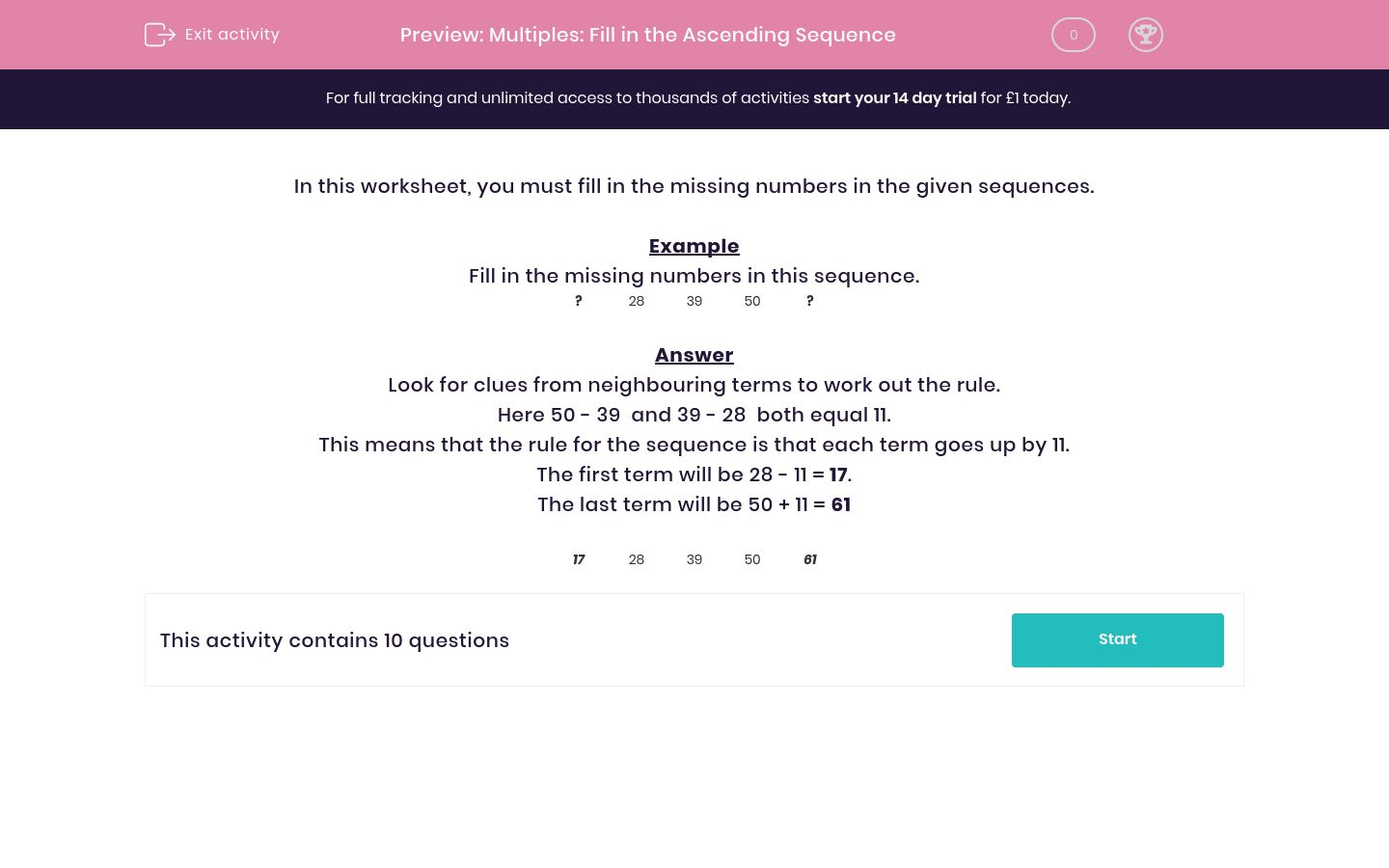# Multiples: Fill in the Ascending Sequence

In this worksheet, students fill in the missing numbers of a sequence by first working out the rule.Key stage:  KS 2

Curriculum topic:   Maths and Numerical Reasoning

Curriculum subtopic:   Factors and Multiples

Difficulty level:### QUESTION 1 of 10

In this worksheet, you must fill in the missing numbers in the given sequences.

Example

Fill in the missing numbers in this sequence.

 ? 28 39 50 ?

Look for clues from neighbouring terms to work out the rule.

Here 50 - 39  and 39 - 28  both equal 11.

This means that the rule for the sequence is that each term goes up by 11.

The first term will be 28 - 11 = 17.

The last term will be 50 + 11 = 61

 17 28 39 50 61
---- OR ----